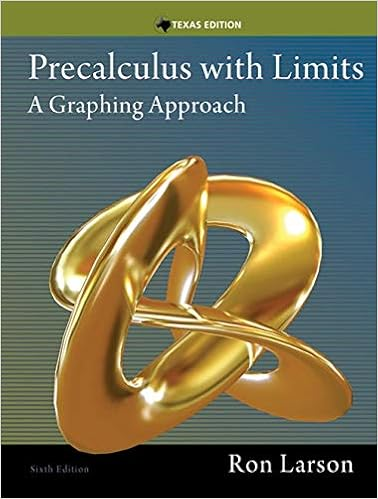# Figure 1 radiation sensor the spectral response of

• Notes
• 41
• 100% (5) 5 out of 5 people found this document helpful

This preview shows page 15 - 19 out of 41 pages.

##### We have textbook solutions for you!
The document you are viewing contains questions related to this textbook.The document you are viewing contains questions related to this textbook.
Chapter 3 / Exercise 109
Precalculus with Limits: A Graphing Approach
LarsonExpert Verified
sensing element, a miniature thermopile, produces a voltage proportional to the intensity of the radiation. Figure 1. Radiation Sensor The spectral response of the thermopile is essentially flat in the infrared region (from 0.5 to 40 pm), and the voltages produced range from the microvolt range up to around 100 millivolts. (A good millivolt meter is sufficient for all the experiments described in this manual.)
##### We have textbook solutions for you!
The document you are viewing contains questions related to this textbook.The document you are viewing contains questions related to this textbook.
Chapter 3 / Exercise 109
Precalculus with Limits: A Graphing Approach
LarsonExpert Verified
13
Specifications: Temperature Range: -65 to 85 °C. Maximum Incident Power: 0.1 Watts/cm2. Spectral Response: .6 to 30pm. Signal Output: Linear from 10-6 to 10-1 Watts/cm2. b) Thermal Radiation Cube (Leslie's Cube) The TD-8554A Radiation Cube (Figure 2) provides four different radiating surfaces that can be heated from room temperature to approximately 120 °C. The cube is heated by a 100 watt light bulb. Just plug in the power cord, flip the toggle switch to "ON", then turn the knob clockwise to vary the power. Measure the cube temperature using the THERMISTOR. The thermistor is embedded in one corner of the cube. Measure the resistance (Figure 1.2), and then use Table 1, below, to translate the resistance reading into a temperature measurement. An abbreviated version of this table is printed on the base of the Radiation Cube. Figure 2 Radiation Cube (Leslie's Cube)
14 Table 1 EQUIPMENT NEEDED:Radiation Sensor, Thermal Radiation Cube — MillivoltmeterNOTES: • If lab time is short, it's helpful to preheat the cube at a setting of 5.0 for 20 minutes before the laboratory period begins. • Part 1 and 2 of this experiment can be performed simultaneously. Make the measurements in Part 2 while waiting for the Radiation Cube to reach thermal equilibrium at each of the settings in Part 1. • When using the Radiation Sensor, always shield it from the hot object except for the few seconds it takes to actually make the measurement. This prevents heating of the thermopile which will change the reference temperature and alter the reading.
15 Radiation Rates from Different Surfaces Part 1 The setup is as shown in Figure 1.1. • Turn on the Thermal Radiation Cube and set the power switch to “HIGH”. Keep an eye on the ohmmeter reading. When it gets down to about 40 kQ, reset the power switch to 5.0. (If the cube is preheated, just set the switch to 5.0.) • When the cube reaches thermal equilibrium-the ohmmeter reading will fluctuate around a relatively fixed
•••